##### Search### SECTION I (50 Marks)

Answer all questions in this section
1.

Use reciprocals, squares and square root tables to evaluate to four significant figures;-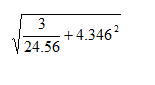4 marks

2.

Find the area of the quadrilateral.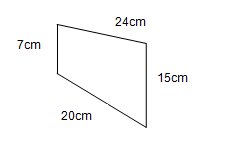4 marks

3.

Wekesa earns a basic salary of Ksh.10,000 per month plus commission of 4% on all sales above Ksh.50, 000. Find his income for a month in which he sold goods of value Ksh.90, 000.

3 marks

4.

Find all the integral values that satisfy the inequality 2x + 3 > 5x - 3 > -8.

4 marks

5.

The angles of elevation of the top of a cliff from two boats A and B on the same side of the cliff on the same horizontal level with the foot of the cliff are 30° and 50° respectively. If the distance from the foot of the cliff to boat B is 30m, find the distance between A and 8.

4 marks

6.

A proper fraction is such that its numerator and denominator have a difference of 2. One is added to the denominator and three subtracted from the numerator, the fraction becomes 2/3. Find the fraction.

3 marks

7.

Simplify the expression;-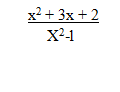3 marks

8.

Evaluate;-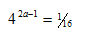3 marks

9.

In the figure below, find the size of angle DCB.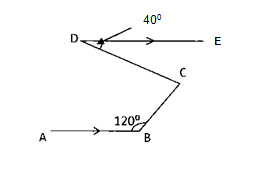3 marks

10.

Find the centre and radius of the circle whose equation is 4x2-4y2 -104y + 6x = 0.

3 marks

11.

The mean mass of four students was 49.5kg. When the masses of another student and a teacher were included, the mean mass became 53kg. If the mass of the student was 16kg less than that of the teacher, what was the teacher's mass?

3 marks

12.

A translation maps the point P (5,-3) onto P' (2,-5); a) Determine the translation vector T. (1MK)

b) A point R' is the image of (-2,-3) under the same translation. Find the magnitude of P'R. (2mks)

3 marks

13.

A chord in the circle of radius 2.8cm subtends an angle of 35° at the circumference of the circle as shown below. Calculate the area of the shaded region.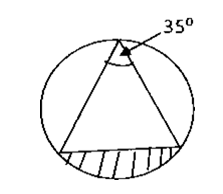3 marks

14.

Two similar right angled triangles have areas of 36cm2 and 9cm2 respectively. If the base of the smaller triangle is 2cm, find the height of the bigger triangle

3 marks

15.

The diagram below shows a histogram representing marks obtained in mathematics test;-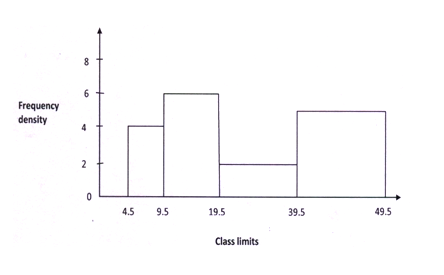From the histogram, develop a frequency distribution table.

2 marks

16.

The interior angles of a hexagon are x+5, 4y-5, 2x+5, 3x, 3x - 20 and 2x. Find the value of x.

2 marks

### SECTION II (50 Marks)

Answer any FIVE questions in this section
17.

Nekesa planned to use sh. 16,800 to buy some text books. When she went to the bookshop, she discovered that the price of the books had increased by sh.200 per book. She could now afford to buy two books less than she had desired to buy with the same amount of money,

a) Determine the number of books she had planned to buy. (6mks)

b) She later sold the books at sh.750 each. Find the percentage profit she made. (4mks)

10 marks

18.

School A is 250km on a bearing of 040° from school B. School C is 200km from A and due East of school B. A fourth school D is on a bearing of 220° from C and due South of school B.
a) Using a scale of 1cm represents 50km; draw an accurate scale drawing showing the positions of schools A, B, C and D. (5mks)

b) By measuring from the scale drawing in (a) above, determine;-

i) The distance BC (1mk)

ii) The distance BD (1mk)

iii) The distance CD (1mk)

iv) The bearing of A from C ( 2mks)

10 marks

19.

The table below shows marks scored by 50 Form four students in mathematics CAT.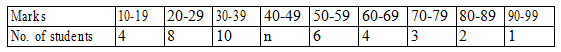a) Find the value of n in the table. (1mk)

b) State the limits of the modal class. (1mk)

c) Calculate the median. (3mks)
d) Calculate the mean of the data (5mks)

10 marks

20.

Easy Coach bus left Bungoma at 10.45am towards Nairobi moving at an average speed of 60km/hr. A shuttle left Bungoma at 1.15pm on the same road to Nairobi at an average speed of 100km/hr, the distance between Bungoma and Nairobi is 400km.
a) Determine the time of the day when the shuttle overtook the bus. (6mks)

b) Both vehicles moved non-stop to Nairobi. Find how long the shuttle waited for the bus to arrive. (4mks)

10 marks

21.

On the grid provided;-a) Draw triangle XYZ whose coordinates are X (8, 6), Y (6, 10) and Z (10, 12) and its image is X'Y'Z' after undergoing a reflection in the line y=x. (4mks)

b) Triangle X'Y'Z' undergoes an enlargement centre (0, 0) scale factor 1/2 to form triangle X"Y"Z". Draw X"Y"Z". (3mks)

c) Triangle X"Y"Z" is rotated about (2, -1) through angle of negative ninty to X'"Y'"Z'". state the coordinates of X'"Y'"Z'". (3mks)

10 marks

22.

In the figure below, OABC is a trapezium. AB is parallel to OC and OC = 5AB D is a point on OC such that OD: DC=3:2.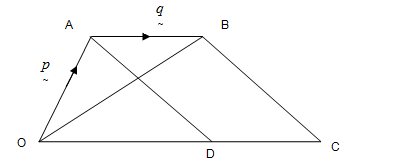a) Given that OA=p and AB=q, express in terms of p and q;-

i) OB (1mk)
ii) AB (2mks)
iii) CD (2mks)

b) Lines OB and AD intersect at point x such that AX=kAD and OX=rOB, where k and r are scalers. Determine the values of k and r.

10 marks

23.

The figure below represents a rectangle PQRS inscribed in a circle with centre O.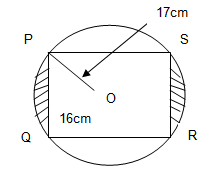Given that the radius OP=17cm and PQ=16cm, calculate;-

a) The length QR of the rectangle. (3mks)

b) The angle ROS. (3mks)

c) The area of the shaded region. (4mks)

10 marks

24.

Wafula bought 3 brands of rice A, B and C. The cost prices were sh.25, sh.30 and sh.45 per kilogram respectively. He mixed the three in the ratio 5:2:1 respectively. After selling the mixture, he made a profit of 20%.

a) How much profit did he make per kilogram of the mixture? (4mks)

b) After one year, the cost price of each brand was increased by 12%.

i) For how much did he sell one kilogram of the mixture to make a profit of 20%. (3mks)

ii) What would have been his percentage profit if he sold one kilogram of the mixture at sh.40.25? (3mks)

10 marks

Back Top